## How To Draw The Cantor Set In Google Sheets

The Cantor set is a special set of numbers lying between 0 and 1, with some fascinating properties.

It’s created by removing the middle third of a line segment and repeating ad infinitum with the remaining segments, as shown in this gif of the first 7 iterations: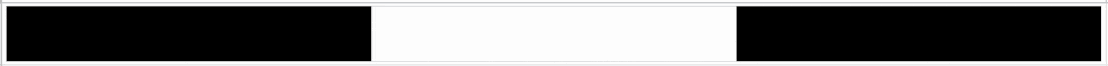The formulas used to create the data for the Cantor set in Google Sheets are interesting, so it’s worth exploring for that reason alone, even if you’re not interested in the underlying mathematical concepts.

But let’s begin by understanding the set in more detail…

## What Is The Cantor Set?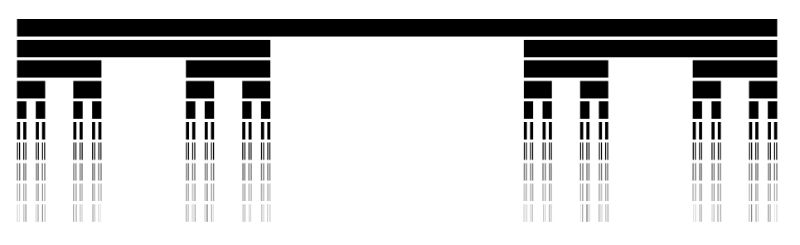The Cantor set was discovered in 1874 by Henry John Stephen Smith and subsequently named after German mathematician Georg Cantor.

The construction shown in this post is called the Cantor ternary set, built by removing the middle third of a line segment and repeating ad infinitum with the remaining segments.

It is sometimes known as Cantor dust on account of the dust of points that remain after repeatedly removing the middle thirds. (Cantor dust also refers to the multi-dimensional version of the Cantor set.)

The set has some fascinating, counter-intuitive properties:

• It is uncountable. That is, there are as many points left behind as there were to begin with.
• It’s self-similar, meaning each subset looks like the whole set.
• It’s fractal with a dimension that is not an integer.
• It has an infinite number of points but a total length of 0.

Wow!

## How To Draw The Cantor Set In Google Sheets

### Create The Data

Step 1:

In a blank sheet called “Data”, type the number “1” into cell A1.

Step 2:

In cell B1, type this formula:

```={ FILTER(A1:A,A1:A<>"") ; SUM(FILTER(A1:A,A1:A<>"")) ; FILTER(A1:A,A1:A<>"") }```

Step 3:

Drag this across your sheet up to column J, which creates the data for the first 10 iterations.

Each formula references the column to the left. For example, the formula in cell D will reference column C.

Your data will look like this: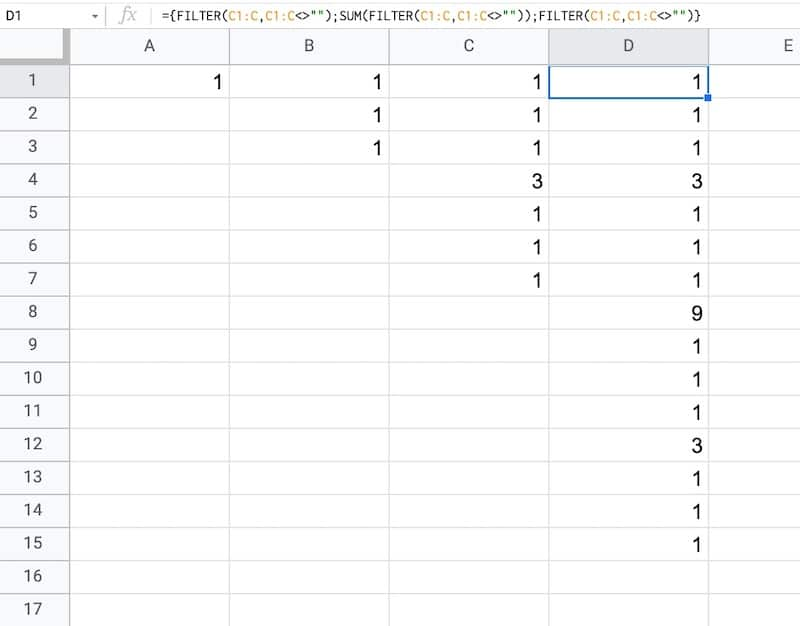### How does this formula work?

It combines array literals and the FILTER function.

Let’s break it down, using the onion framework.

The innermost formula is:

`=FILTER(A1:A,A1:A<>"")`

This formula grabs all the data from column A and returns any non-blank entries, in this case just the value “1”.

Now we combine two of these together with array literals:

```={ FILTER(A1:A,A1:A<>"") ; FILTER(A1:A,A1:A<>"") }```

Here the array literals `{ ... ; ... }` stack these two ranges.

In this first example, it puts the number “1” with another “1” beneath it in column B.

Then we add a third FILTER and also SUM the middle FILTER range to create our final Cantor set algorithm:

```={ FILTER(A1:A,A1:A<>"") ; SUM(FILTER(A1:A,A1:A<>"")) ; FILTER(A1:A,A1:A<>"") }```

As we drag this formula to adjacent columns, the relative column references will change so that it always references the preceding column.

In column B, the output is:

1,1,1

Then in column C, we get:

1,1,1,3,1,1,1

And in column D:

1,1,1,3,1,1,1,9,1,1,1,3,1,1,1

etc.

This data is used in the sparkline to generate the correct gaps for the Cantor set.

### Draw The Cantor Set

We’ll use sparklines to draw the Cantor set in Google Sheets.

Step 4:

Create a new blank sheet and call it “Cantor Set”.

Step 5:

Next, create a label in column A to show what iteration we’re on.

Put this formula in cell A1 and copy down the column to row 10:

`="Cantor Set "&ROW()`

This creates a string, e.g. “Cantor Set 1”, where the number is equal to the row number we’re on.

Step 6:

The next step is to dynamically generate the range reference. As we drag our formula down column B, we want this formula to travel across the row in the “Data” tab to get the correct data for this iteration of the Cantor set.

Start by generating the row number for each row with this formula in cell B1 and copy down the column:

`=ROW()`

(I set up my sheet with the data in columns because it’s easier to create and read that way. But then I want the Cantor set in a column too, hence why I need to do this step.)

Step 7:

Use the row number to generate the corresponding column letter with this formula in cell C1 and copy down the column:

`=ADDRESS(1,ROW(),4)`

This uses the ADDRESS function to return the cell reference as a string.

Step 8:

Remove the row number with this formula in cell D1 and copy down the column:

`=SUBSTITUTE(ADDRESS(1,ROW(),4),"1","")`

Step 9:

Combine these two references to create an open-ended range reference for the correct column of data in the “Data” sheet.

Put this formula in cell E1 and copy down the column:

`="'Data'!"&ADDRESS(1,ROW(),4)&":"&SUBSTITUTE(ADDRESS(1,ROW(),4),"1","")`

This returns range references e.g. `'Data'!A1:A`

Step 10:

Put this formula in cell F1 and copy down the column:

`=INDIRECT("'Data'!"&ADDRESS(1,ROW(),4)&":"&SUBSTITUTE(ADDRESS(1,ROW(),4),"1",""))`

This will show #REF! errors: “Array result was not expanded because it would overwrite data in…”

However, don’t worry, these are only temporary as we’ll dump this data into the sparkline formula next.

Step 11:

In column G, create a default sparkline formula:

`=SPARKLINE(INDIRECT("'Data'!"&ADDRESS(1,ROW(),4)&":"&SUBSTITUTE(ADDRESS(1,ROW(),4),"1","")))`

This shows the default line chart (except for the first row where it shows a #N/A error).

Step 12:

In column H, convert the line chart sparkline to a bar chart sparkline by specifying the charttype in custom options:

`=SPARKLINE(INDIRECT("'Data'!"&ADDRESS(1,ROW(),4)&":"&SUBSTITUTE(ADDRESS(1,ROW(),4),"1","")),{"charttype","bar"})`

Step 13 (optional):

Finally, in column I, change the colors to a simple black and white scheme, by specifying color1 and color2 inside the sparkline:

`=SPARKLINE(INDIRECT("'Data'!"&ADDRESS(1,ROW(),4)&":"&SUBSTITUTE(ADDRESS(1,ROW(),4),"1","")),{"charttype","bar";"color1","black";"color2","white"})`

Feel free to delete any working columns once you have finished the formula showing the Cantor set.

## Finished Cantor Set In Google Sheets

Here are the first 10 iterations of the algorithm to create the Cantor set:

Of course, this is a simplified representation of the Cantor set. It’s impossible to create the actual set in a Google Sheet since we can’t perform an infinite number of iterations.

## Can I see an example worksheet?

Yes, here you go.

You might enjoy my other mathematical Google Sheet posts:

PI Function in Google Sheets And Other Fun π Facts

How To Draw The MandelBrot Set In Google Sheets, Using Only Formulas

The FACT Function in Google Sheets (And Why A Shuffled Deck of Cards Is Unique)

In this post, I’m going to show you how to create radial bar charts in Google Sheets.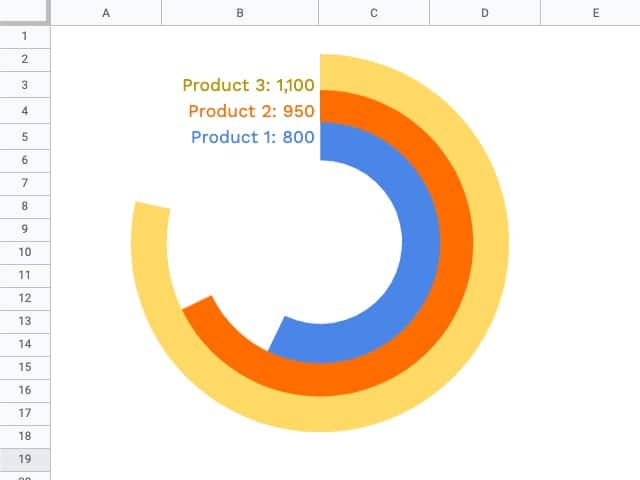They look great and grab your attention, which is important in this era of information overload.

But they should be used sparingly because they’re harder to read than a regular bar chart (because it’s harder to compare the length of the curved bars).

Let’s begin with the data.

In this example, we’ll create a radial bar chart in Google Sheets with 3 series.

We need a column of values for these 3 series, for example, products with a number of units sold.

Next, we need some upper limit (max value) for our bars. This allows us to scale the bars properly.

Lastly, we need a helper column that calculates the difference between the max value and the actual value.

Here’s the data for the radial bar chart, in cells `E3:H6`: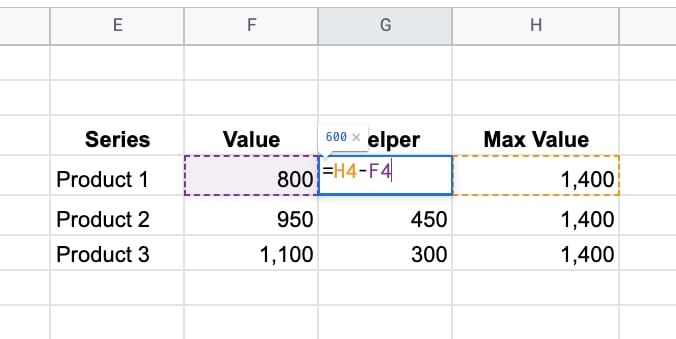Ok, I’m going to let you in on a little secret now…

This is not a single chart. No sir, it’s three charts overlaid on top of each other.

And yes, this means it takes three times as long to create!

### Step 1: Create the inner circle

Highlight the first row of data but exclude the max value column. In the example dataset above, highlight `E4:G4` and insert a chart.

Select a doughnut chart.

Under the Setup menu, make sure to check the “Switch rows/columns” checkbox, so your chart looks like this: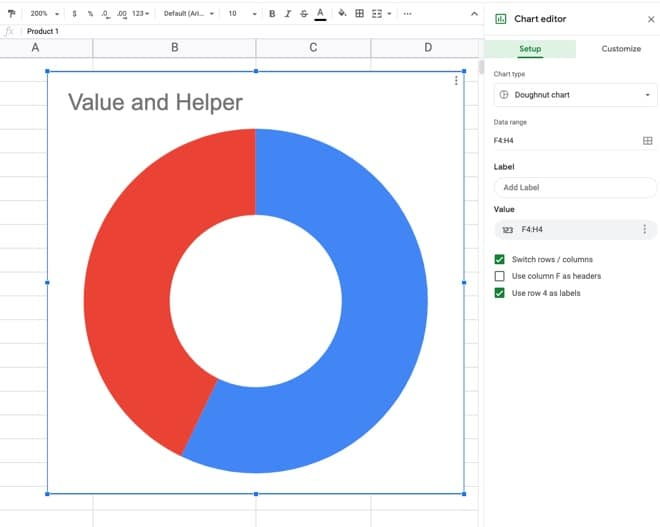Under the customize menu of the chart tool, set the following conditions:

• Background color: None
• Chart border color: None
• Donut hole size: 67%
• Set Slice 2 color to none
• Remove the chart title
• Set the legend to none

This is what the inner donut should look like: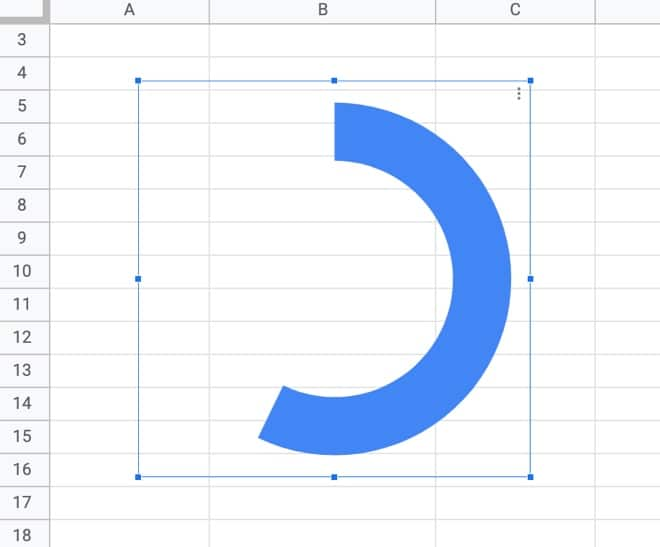### Step 2: Create the middle circle

Repeat the steps above for the inner circle, but use the next row of data, choose a different color, and set the donut hole size to 77% (you may have to experiment with these percentages to line everything up at the end).

Drag the second donut chart on top of the first and line up the radial bars to get: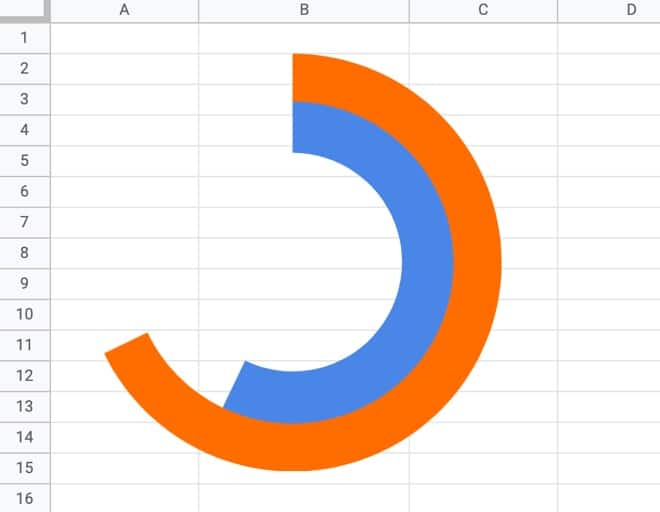### Step 3: Create the outer circle

Again, repeat the steps above from the inner circle to create a third donut chart, using the third row of data, a different color, and setting the donut hole size to 81% (again, this might need tweaking to line everything up).

Drag this third donut chart on top of the other two and you have a radial bar chart in Google Sheets!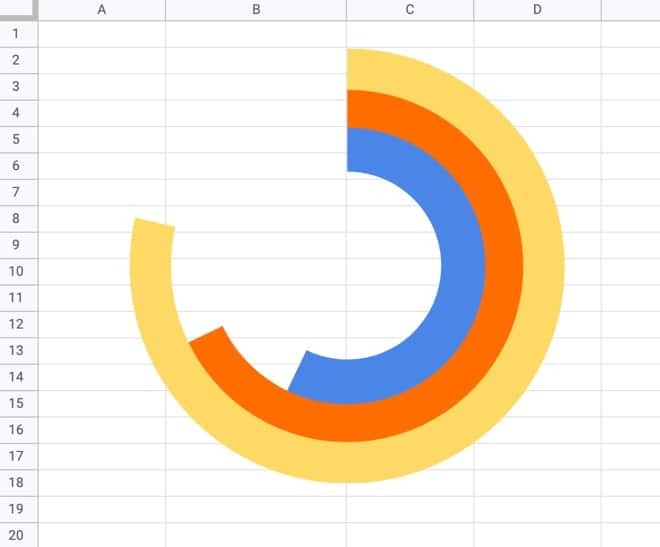Note on editing charts:

Since the charts are placed on top of each other, you’ll only be able to access the top chart to edit. You’ll have to move it to the side to access the chart underneath, and then move that one if you want to access the inner chart.

### Step 4: Add the data labels

It gets messy to add the data labels to each chart through the chart editor, so I opted to create formulas to add my data labels into the cells next to each bar of the radial bar chart.

To access cells underneath the charts, click on a cell outside of the chart area and then use the arrow keys on your keyboard to reach the desired cell.

Once there, add the following formula:

`=E6&": "&TEXT(F6,"#,0")`

This formula uses the TEXT function to combine text and numbers in Google Sheets.

This shows the series name and value alongside each bar: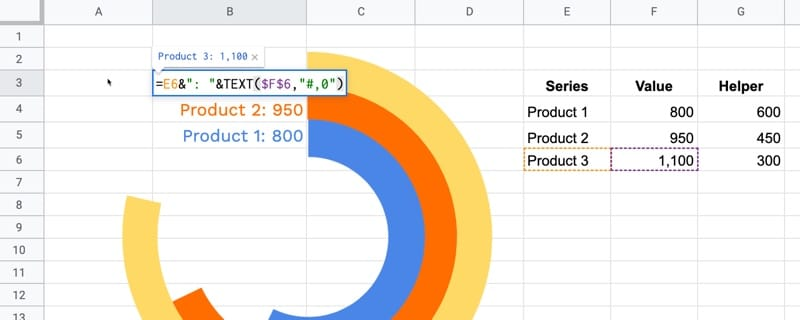To finish, remove the gridlines from your Sheet to give the chart a clean look.## Can I see an example worksheet for the radial bar chart?

Yes, here you go.

## Real World Examples of Radial Bar Charts

My friend Jeff Sauer, who founded Data Driven U to teach people data-driven marketing, contacted me recently about creating a radial bar chart for one of his workshops.

He is graciously sharing his report here, so you can see a radial bar chart with six rings:This is a screenshot of his Google Sheet!

(If you’re looking for top draw digital marketing, then you should definitely check out Jeff’s site: DataDrivenU.com This is not an affiliate link, just a personal recommendation!)

You’ve probably also seen a radial bar chart in the wild with the Apple Watch Rings Chart!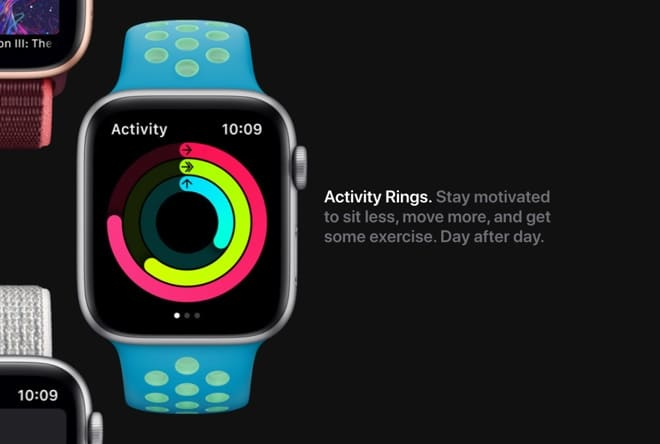This post explores the Google Sheets REGEX formulas with a series of examples to illustrate how they work.

Regular expressions, or REGEX for short, are tools for solving problems with text strings. They work by matching patterns.

You use REGEX to solve problems like finding names or telephone numbers in data, validating email addresses, extracting URLs, renaming filenames containing the word “Application” etc.

They have a reputation for being hard, but once you learn a few basic rules and understand how they work you can use them effectively.

There are three Google Sheets REGEX formulas: REGEXMATCH, REGEXEXTRACT, and REGEXREPLACE. Each has a specific job:

REGEXMATCH will confirm whether it finds the pattern in the text.

REGEXEXTRACT will extract text that matches the pattern.

REGEXREPLACE will replace text that matches the pattern.

Let’s understand them with a series of examples.

## Example 1: Google Sheets REGEX Formula REGEXMATCH

The REGEXMATCH function returns a TRUE if it matches the pattern you provide anywhere in the text and FALSE if there are no matches in the text.

For example, suppose we have this dataset of vintage wines, where each row has a mix of text and/or numbers: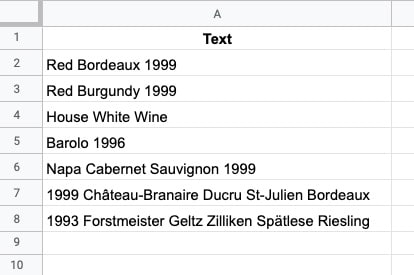Let’s create a simple REGEXMATCH to test whether a cell contains a number, i.e. the year vintage is given.

In cell A2, add this formula:

`=REGEXMATCH(A2,"[0-9]")`

This will give a TRUE output if it finds a number in the string, or FALSE if there are no numbers.

`[0-9]+` matches any number from 0 to 9 in the input string.

So, provided there is one number in the input string, this pattern will give us a match: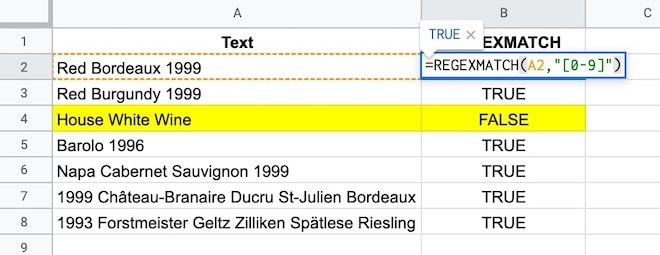See how the text without any numbers “House white wine” gives a FALSE output because there is no match.

### Important Note

This pattern matches a single number. It doesn’t care what else might be in the cell.

For example, it returns TRUE for a meaningless string containing numbers, which is probably not the behavior you’re looking for in this case.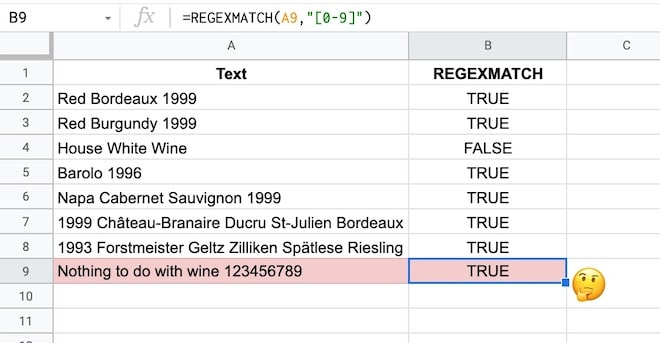By the end of this tutorial, you’ll understand enough to know how to fix this yourself.

(For the solution, see the formula at the end of this tutorial.)

## Example 2: Google Sheets REGEX Formula REGEXEXTRACT

Using the same wine dataset as above, we want to create a new column in our dataset with the vintages, i.e. a column with the year only.

This is a perfect example of when to use a Google Sheets REGEX formula. We’ll create a regular expression pattern to match any numbers and then use REGEXEXTRACT to extract them.

As with everything in spreadsheets, there are multiple REGEX patterns that could solve this.

We saw the `[0-9]` pattern above, but we can also use the named character class for digits `\d`

This matches any digits (i.e. numbers 0 to 9).

So the REGEXEXTRACT formula to extract the year looks like this:

`=REGEXEXTRACT(A2,"[0-9]+")`

or

`=REGEXEXTRACT(A2,"\d+")`

The `+` means get one or more.

Both formulas return a result of “1999”, because the text in A2 is “Red Bordeaux 1999”.

If no numbers are found, the formula returns a #N/A error.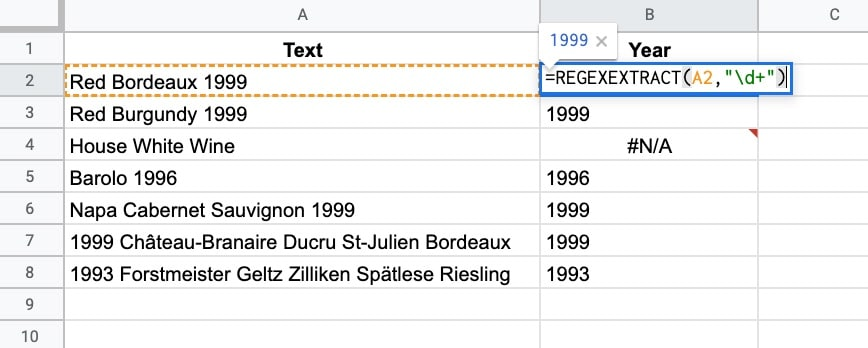### Two Important Notes

Note 1:

The REGEX formulas require text inputs and they give you text outputs back. So the 1999 output above is formatted as text. To convert to a number you need to wrap the result with a VALUE function. See Example 4 below for more details.

Note 2:

If there were more numbers in the text string e.g. “Red Bordeaux 1999 or 2001” only 1999 is returned by the REGEXEXTRACT formula because it doesn’t match the space or letters between the numbers.

It only matches the numbers, so it matches the first number it sees, then keeps matching numbers until it hits the first non-number where it stops matching, i.e. the space at the end of 1999.

## Example 3: Google Sheets REGEX Formula REGEXREPLACE

The REGEXREPLACE will replace all sets of numbers in the text with a new value, for example, this formula:

`=REGEXREPLACE(A2,"\d+","2021")`

will replace 1999 in the sentence “Red Bordeaux 1999” with “2021” and return the answer: Red Bordeaux 2021.

### Important Note

The REGEXREPLACE function replaces ALL sets of numbers in the text, unlike the REGEXEXTRACT which just extracts the first pattern it matches.

## Example 4: Use REGEXEXTRACT And VALUE To Extract Numbers From Text

Consider this data about a supertanker ship: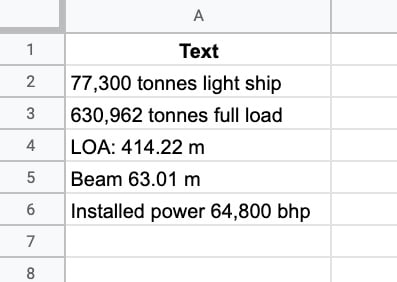Is it possible to extract those numbers with a REGEX formula, even though they’re formatted differently?

You bet!

This formula will extract numbers with or without thousand separators and/or decimal places:

`=REGEXEXTRACT(A2,"[\d,.]+")`

The REGEX pattern `[\d,.]+` means match any digits, commas, or periods and extract them.

So the REGEXEXTRACT formula matches the first digit it finds then keeps going with the match provided the next character is either another digit or a comma or a period, When it reaches something else, like a space or a letter, the match breaks, and the extract is completed.

We’re not quite done yet though.

### Using The VALUE Function To Convert The Output To Numbers

The output of the REGEXEXTRACT formula is a string, not a number.

So we need to convert the output to a number by wrapping the result with the VALUE function like this:

`=VALUE(REGEXEXTRACT(A2,"[\d,.]+"))`

The formula above is not foolproof, however.

### Improving The Pattern Match

If the text string has a period or comma before the first digit then this will be extracted as the match.

For example, if the input text string was: “The ship is huge. It’s 630,962 tonnes full load.” then the REGEXEXTRACT formula from above will extract the first period only.

It matches the period after “huge” and then stops the match because of the space character that follows.

How do we modify the formula to ensure the extract begins with a number?

Well, we change the REGEX to match a number first, before anything else, like this:

`=REGEXEXTRACT(A2,"\d[\d,.]*")`

Here the REGEX matches on a digit first, before looking for more digits, commas, or periods.

If you’re eagle-eyed you’ll notice that the plus `+` has changed to a star `*` after the square bracket. This means zero or more of the characters in the square brackets, to account for a situation where there is a single-digit number that we want to match.

The REGEX pattern `\d[\d,.]*` matches a digit, followed by zero or more characters that are digits, commas, or periods.

Now the result of the formula extraction is 630,962, which is the correct answer.

Remember, the output of the REGEXEXTRACT formula is a string, so you’ll need to wrap it with the VALUE function to convert it to a number, e.g.

`=VALUE(REGEXEXTRACT(A2,"\d[\d,.]*"))`

## Example 5: Check Telephone Numbers With REGEXMATCH

For this example, I’m going to consider US phone numbers with dashes between the sections, i.e. numbers of this format: XXX-XXX-XXXX

It’s 3 digits, then a dash, 3 digits, dash, then 4 digits.

By the end of this tutorial, you’ll have enough information to modify the example to other regions of the world.

Here’s the data: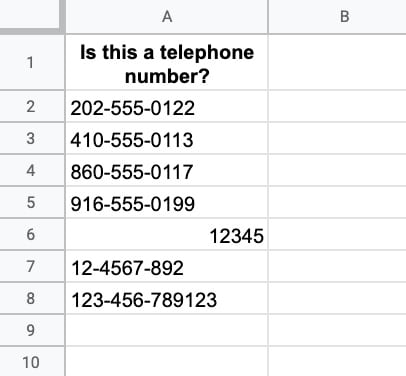Let’s build a REGEX formula to check whether the string in column A is a valid US phone number.

Using what we learned above, we know that the expression `\d` matches digits. So our first attempt is this formula:

Step 1:

`=REGEXMATCH(A2,"\d\d\d-\d\d\d-\d\d\d\d")`

This matches 3 digits, then a dash, 3 digits, dash, then 4 digits and it works ok. It shows TRUE when it matches a telephone number and FALSE otherwise.

But it’s verbose. We can simplify it by using a quantifier clause.

Step 2:

`=REGEXMATCH(A2,"\d{3}-\d{3}-\d{4}")`

The `{3}` means match exactly 3 of the preceding pattern, i.e. match exactly 3 digits.

This works great, except it still matches the final number on row 8. It matches the 3-3-4 and doesn’t care about the extra digits that come at the end.

But we know this isn’t a valid phone number, so how do we get it to stop after the 4 digits and discount anything with more than 4 digits in the final set?

### Word Boundaries

We wrap the expression with a special expression called a word boundary, denoted by `\b` before and after the main expression.

Technically, this matches a “zero-width nothing”. What that means is that it marks a boundary between a word character (e.g. letter or digit or _) and a non-word character. So it will match the digits up to 4 and then look for a boundary. A fifth digit will break the match, but a space won’t because it defines a boundary.

Our new expression looks like this, with a \b at the beginning and end.

Step 3:

`=REGEXMATCH(A2,"\b\d{3}-\d{3}-\d{4}\b")`

The final thing we might do is to wrap this with an IFERROR function to handle number inputs like row 6 above that cause an error output (since the REGEX formulas only work with text strings).

Step 4:

`=IFERROR(REGEXMATCH(A2,"\b\d{3}-\d{3}-\d{4}\b"),FALSE)`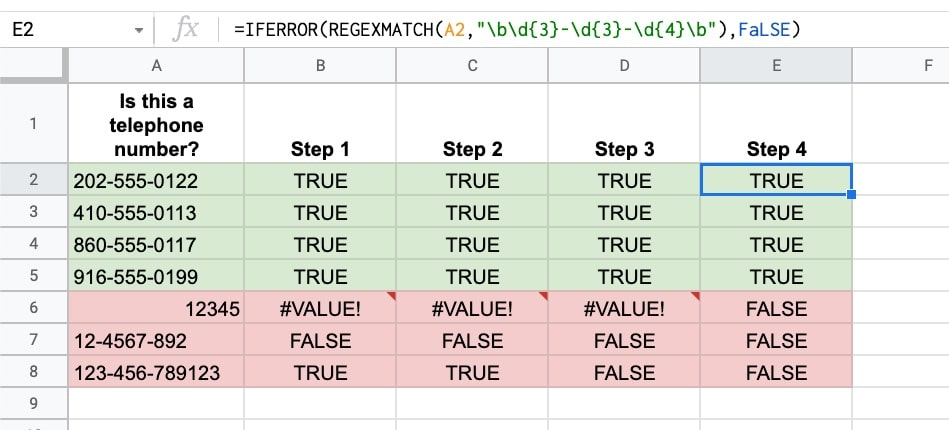## Example 6: Reorder Name Strings With REGEXREPLACE

In this example, we’re looking at the REGEXREPLACE function and a key concept in regular expressions: capturing groups.

Suppose we have a list of names in this format: “Surname, First Name” and we want to switch the order to “First Name Surname”.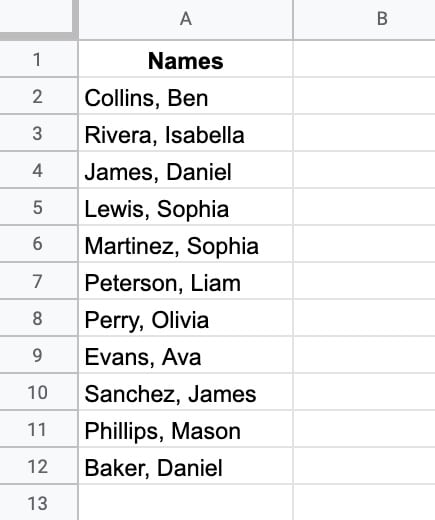Here’s the formula to switch the order of the names:

`=REGEXREPLACE(A2,"(\w+)(?:, )(\w+)","\$2 \$1")`

Let’s break this down:

REGEXREPLACE finds all substrings that match the pattern and replaces them with the value given. It takes 3 arguments: 1) the input text, 2) the pattern to match, and 3) the replacement value.

Let’s look at each in turn:

### Input text

Surname, First name e.g. Collins, Ben

### Matching pattern

`(\w+)(?:, )(\w+)`

`\w+` matches word characters

`(\w+)` creates a numbered capturing group. It matches the first word up to the comma.

`(?:` opens a non-capturing group, which essentially means match but don’t capture the text in this group

`(?:, )` is the non-capturing group that matches a comma and space but doesn’t capture them

`(\w+)` creates a second numbered capturing group. It matches the second word after the comma and space.

### Replacement Value

`\$2 \$1`

Now, this is where it gets interesting!

Our matching pattern captured each of the names as a numbered group, which we’re now able to refer to with `\$1` or `\$2`

Group `\$1` captured the surname and group `\$2` captured the first name.

To reverse the names, we put group `\$2` first, then `\$1`

The output looks like this: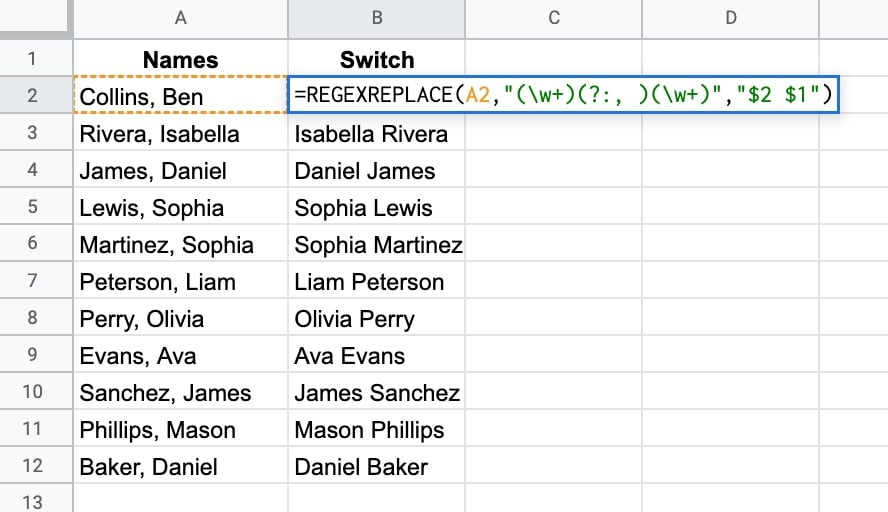## SOLUTION From Example 1

Modify the REGEXMATCH formula to be:

`=REGEXMATCH(A2,"\b[0-9]{4}\b")`

It uses a quantifier `{4}` and word boundaries `\b` to only match 4-digit numbers (see example 5 for more information on quantifiers and word boundaries).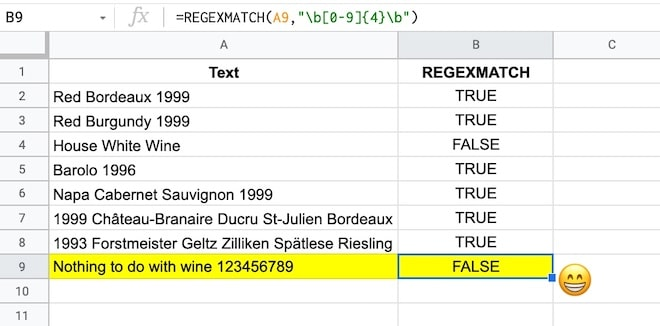### The Google Sheets REGEX Formula Cookbook

There are hundreds of more REGEX examples in my Google Sheets REGEX Formula Cookbook course.

### Resources

For the full list of allowed syntax, check the re2 syntax page.

## The FACT Function in Google Sheets (And Why A Shuffled Deck of Cards Is Unique)

Let’s start with a mind-blowing fact, and then use the FACT function in Google Sheets to explain it.

Pick up a standard 52 card deck and give it a good shuffle.

The order of cards in a shuffled deck will be unique.

One that has likely never been seen before in the history of the universe and will likely never be seen again.

I’ll let that sink in.

Isn’t that mind-blowing?

Especially when you picture all the crazy casinos in Las Vegas.

Let’s understand why, and in the process learn about the FACT function and basic combinatorics (the study of counting in mathematics).

## Four Card Deck

To keep things simple, suppose you only have 4 cards in your deck, the four aces.

You can create this deck in Google Sheets with the CHAR function: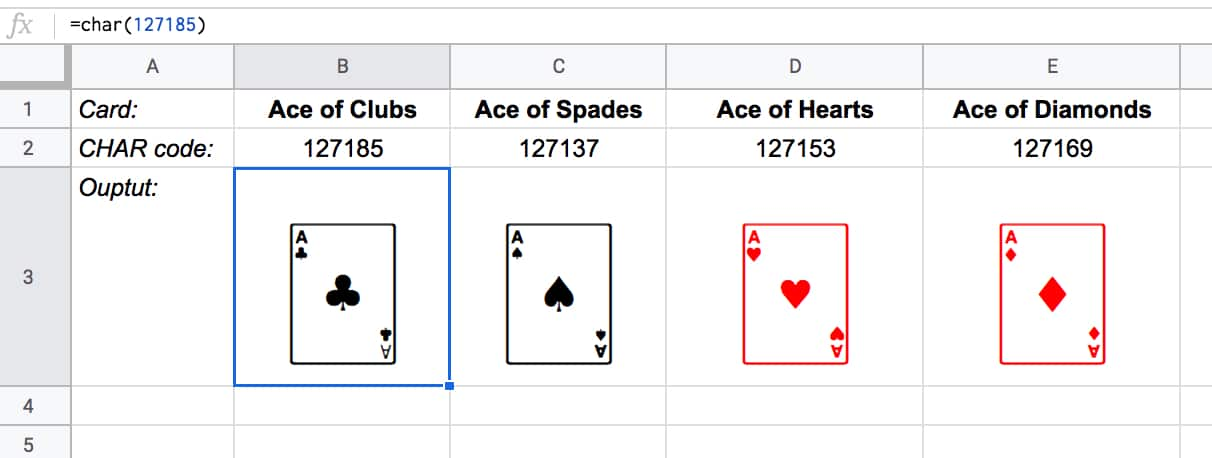The formulas to create these four cards are:

Ace of Clubs:

`=CHAR(127185)`

`=CHAR(127137)`

Ace of Hearts:

`=CHAR(127153)`

Ace of Diamonds:

`=CHAR(127169)`

Let’s see how many different combinations exist with just these four cards.

Pick one of them to start. You have a choice of four cards at this stage.

Once you’ve chosen the first one, you have three cards left, so there are 3 possible options for the second card choice.

When you’ve picked that second card, you have two cards left. So you have a choice of two for the third card.

The final card is the last remaining one.

So you have 4 choices * 3 choices * 2 choices * 1 choice = 4 * 3 * 2 * 1 = 24

There are 24 permutations (variations) with just 4 cards!

Visually, we can show this in our Google Sheet by displaying all the different combinations with the card images from above: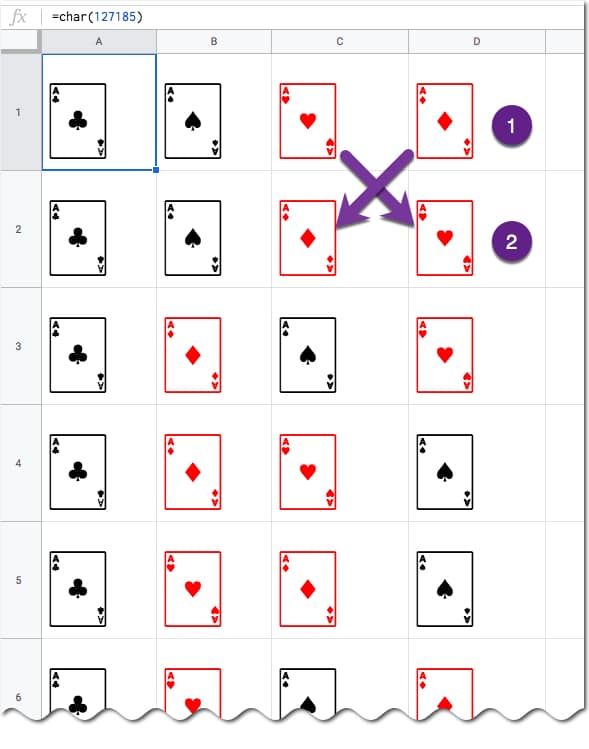(I’ve just shown the first 6 rows for brevity.)

You can see for example, when moving from row 1 to row 2, we swapped the position of the two red suits: the Ace of Hearts and the Ace of Diamonds.

## Five Card Deck

This time there are 5 choices for the first card, then 4, then 3, then 2, and finally 1.

So the number of permutations is 5 * 4 * 3 * 2 * 1 = 120

Already a lot more! I have not drawn this out in a Google Sheet and leave that as an optional exercise for you if you wish.

## The FACT function

The FACT function in Google Sheets (see documentation) is a math function that returns the factorial of a given number. The factorial is the product of that number with all the numbers lower than it down to 1.

In other words, exactly what we’ve done above in the above calculations.

Four:

The 4 card deck formula is:

=FACT(4)

which gives an answer of 24 permutations.

Five:

The 5 card deck formula is:

=FACT(5)

which gives an answer of 120 permutations.

Six:

A 6 card deck is:

=FACT(6)

which gives an answer of 720 permutations.

Twelve:

A 12 card deck has  479,001,600 different ways of being shuffled:

=FACT(12)

(You’re more likely to win the Powerball lottery at 1 in 292 million odds than to get two matching shuffled decks of cards, even with just 12 cards!)

Fifty Two:

Keep going up to a full deck of 52 cards with the formula and it’s a staggeringly large number.

=FACT(52)

Type it into Google Sheets and you’ll see an answer of 8.07E+67, which is 8 followed by 67 zeros!

(This number notation is called scientific notation, where huge numbers are rounded to the first few digits multiplied by a 10 to the power of some number, 67 in this case.)

This answer is more than the number of stars in the universe (about 10 followed by 21 zeros).

Put another way if all 6 billion humans on earth began shuffling cards at 1 deck per minute every day of the year for millions of years, we still wouldn’t even be close to finding all possible combinations.

## Build Numbered Lists With The Amazing SEQUENCE Function

The SEQUENCE function is a useful function in Google Sheets. It’s a powerful way to generate numbered lists.

Previously, you had to resort to obscure array formulas like

`=ArrayFormula(row(1:5))`

to get lists of numbers. Things got ugly fast if you wanted to customize these lists.

Thankfully today, we have the SEQUENCE function.

As arguments, you specify: 1) the number of rows, 2) the number of columns, 3) a start value, and 4) a step size.

Arguments 2, 3 and 4 are optional. However, if you want to set them you need to include the previous ones (e.g. if you want to set a step size in argument 4, then you need to set 1, 2 and 3 as well).

Keep this order in mind as you look through the examples below and you’ll soon understand how the function works.

## 1. Ascending list of numbers

`=SEQUENCE(5)`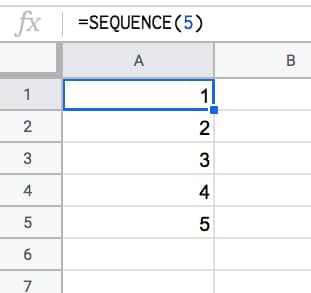## 2. Horizontal list of numbers

Set the row count to 1 and the column count to however many numbers you want e.g. 5:

`=SEQUENCE(1,5)`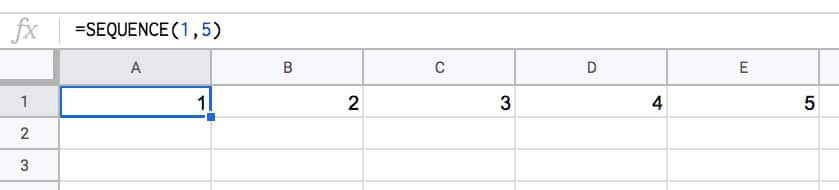## 3. Two-dimensional array of numbers

Set both row and number values:

`=SEQUENCE(10,5)`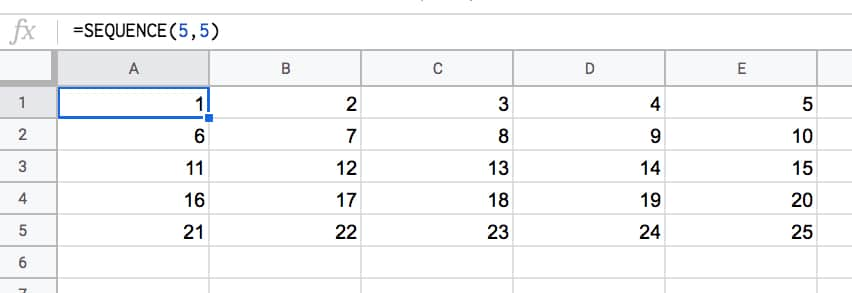## 4. Start from a specific value

Set the third argument to the value you want to start from e.g. 100:

`=SEQUENCE(5,1,100)`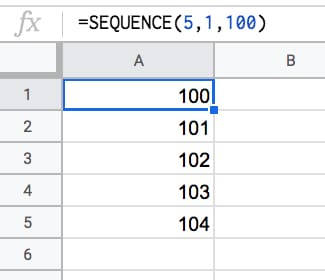## 5. Use a custom step

Set the fourth argument to the size of the step you want to use, e.g. 10:

`=SEQUENCE(5,1,1,10)`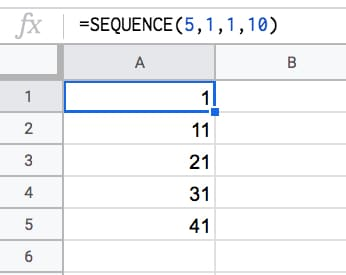## 6. Descending numbers

Set the fourth argument to -1 to count down:

`=SEQUENCE(5,1,5,-1)`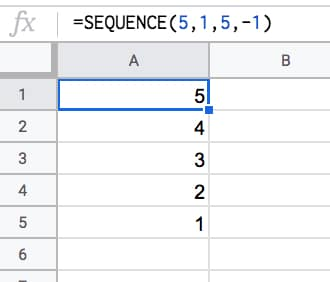## 7. Negative numbers

Set the start value to a negative number and/or count down with negative step:

`=SEQUENCE(5,1,-1,-1)`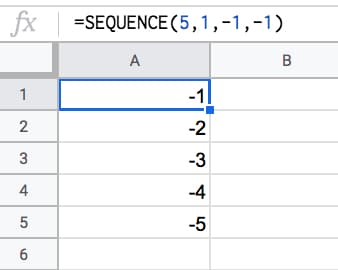## 8. Dates

Dates are stored as numbers in spreadsheets, so you can use them inside the SEQUENCE function. You need to format the column as dates:

`=SEQUENCE(5,1,TODAY(),1)`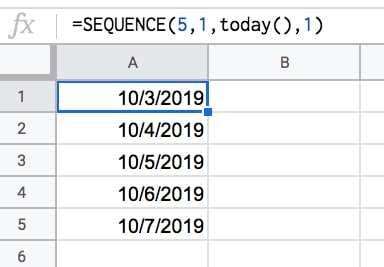## 9. Decimal numbers

Unfortunately you can’t set decimal counts directly inside the SEQUENCE function, so you have to combine with an Array Formula e.g.

`=ArrayFormula( SEQUENCE(5,1,10,1) / 10 )`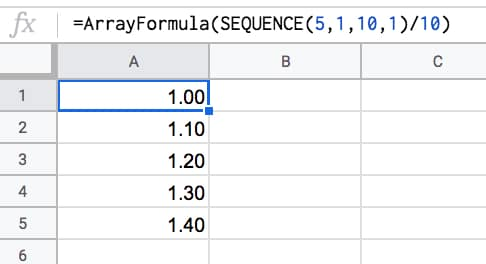## 10. Constant numbers

You’re free to set the step value to 0 if you want an array of constant numbers: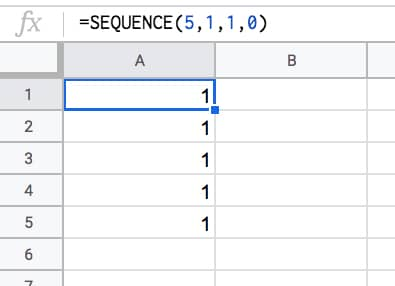## 11. Monthly sequences

Start with this formula in cell A1, which gives the numbers 1 to 12 in a column:

`=SEQUENCE(12)`

In the adjacent column, use this DATE function to create the first day of each month (formula needs to be copied down all 12 rows):

`=DATE(2021,A1,1)`

This can be turned into an Array Formula in the adjacent column, so that a single formula, in cell C1, outputs all 12 dates:

`=ArrayFormula(DATE(2021,A1:A12,1))`

Finally, the original SEQUENCE formula can be nested in place of the range reference, using this formula in cell D1:

`=ArrayFormula(DATE(2021,SEQUENCE(12),1))`

This single formula gives the output:

1/1/2021
2/1/2021
.
.
.
12/1/2021

It’s an elegant way to create a monthly list. It’s not dependent on any other input cells either (columns A, B, C are working columns in this example).

With this formula, you can easily change all the dates, e.g. to 2022.

Building in steps like this a great example of the Onion Method, which I advocate for complex formulas.

## 12. Text and Emoji sequences

You can use a clever trick to set the SEQUENCE output to a blank string using the TEXT function. Then you can append on a text value or an emoji or whatever string you want to create a text list.

For example, this repeats the name “Ben Collins” one hundred times in a column:

`=ArrayFormula(TEXT(SEQUENCE(100,1,1,1),"")&"Ben Collins")`

And, by using the CHAR function, you can also make emoji lists. For example, here’s a 10 by 10 grid of tacos:

`=ArrayFormula(TEXT(SEQUENCE(10,10,1,1),"")&CHAR(127790))`Have you got any examples of using the SEQUENCE function?